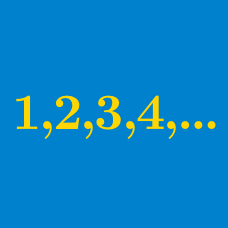Algebra

# Arithmetic Progressions Warmup

If $9, b, c, 18$ is an arithmetic progression, what is the value of $c?$

True or false?

If $(A, B, C, D,...)$ and $(a, b, c, d,...)$ are both arithmetic progressions, then $(A-a, B-b, C-c, D-d, ...)$ is guaranteed to also be an arithmetic progression.

Jaspreet had $5 in her account on Day 1. On each subsequent day after Day 1, she deposited a constant amount into her account. At the end of Day 101, she has$305.

How many dollars did she have in her account at the end of Day 3?

Suppose $a, b, c, d, ...$ is an arithmetic progression with $b = 8.$ What is the average (arithmetic mean) of $a, b,$ and $c?$

$5,9,a,b,c,...$

If this sequence is an arithmetic progression, what is the value of $c?$

×